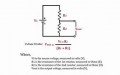#### WakelessFoil

Joined Apr 16, 2020
12
I am trying to use a potentiometer to drop voltage. I just learned about resistors and voltage drop so I thought that if I supply an input voltage I can reduce it using the pot. But when I attach my volt meter on the other side where my load/output would be I get VCC? Can someone help me understand this conceptually? I am not interested in understanding electronics by memorizing rules rather than actually grasping what is going on!

#### ericgibbs

Joined Jan 29, 2010
16,748
hi WF,
You have to consider the impedance/resistance of your Voltmeter.
The 512R and the meter resistance are in series.
E

#### Attachments

•#### WBahn

Joined Mar 31, 2012
27,854
Your voltmeter has a very high (ideally infinite) resistance, so the total current in your circuit is zero, meaning that the voltage drop across your resistor is zero.

Your meter across the resistor is showing 512 nV which, across 512 Ω, is a current of 1 nA. That would indicate a meter resistance in the 1 GΩ range. I'm assuming these numbers are from a simulation of some kind? Typical real meters have resistances in the 10 MΩ range, give or take an order of magnitude.

•#### MrChips

Joined Oct 2, 2009
27,651
The one rule that you must learn and memorise is Ohm's Law.
This is stated as
I = V / R

The two corollaries are
V = I x R
R = V / I

Without this knowledge you are going nowhere fast.
You use Ohm's Law in order to understand how voltage dividers and potentiometers behave.When you apply a load, or even a test meter, you alter the circuit. You need to reapply Ohm's Law while taking into account the added load or test meter.•#### dw85745

Joined Oct 5, 2015
32
WakelessFoil: If you're not familiar with Ohm's triangle, it is a very easy way to remember the formulas. HTH

#### WakelessFoil

Joined Apr 16, 2020
12
Thank you all for the replies.

I am familiar with ohms law. What I don't understand is why in a two resistor series circuit the voltage gets reduced after R1, but when R2 is removed you get 1V where it used to be 500mV. I always thought of voltage as a measurement of potential energy in a certain node (got this from potential difference). But this does not hold up when compared to the single resistor open circuit.

The application for this is that I thought I could control voltage going to a load in a DC circuit using a single potentiometer (variable resistance). I wanted to use the pot to control voltage going into the base of a 2N2222 transistor so I could control the current between the collector and emitter.

#### MrChips

Joined Oct 2, 2009
27,651
Apply Ohm’s Law and study the results.

Edit:
V = I x R
If I = 0, V across the resistor R must be zero.

#### WBahn

Joined Mar 31, 2012
27,854
A resistor can only drop voltage across it when there is current flowing through it.

Imagine taking a water pump connected to a long, thin pipe. When the pump is on and water is flowing through the pipe, there is a significant pressure drop across the pipe. But if you cap the end of the pipe so that no water can flow, the pressure at the end of the pipe will rise to the pump's output pressure.

#### crutschow

Joined Mar 14, 2008
31,097
I am familiar with ohms law.
You questions do not suggest that.
If you were, then you should be able to see that with no (or little) resistor current, there is no (or little) voltage drop.

Last edited:
•panic mode

#### panic mode

Joined Oct 10, 2011
2,360
then make R2 equal to voltmeter input resistance... what do you expect voltage drops across R1 and R2 to be?

#### MrChips

Joined Oct 2, 2009
27,651
I am not interested in understanding electronics by memorizing rules rather than actually grasping what is going on!
I am familiar with ohms law.
Sometimes intuition works. Sometimes it doesn't.
It this case it doesn't. This is where you do have to study Ohm's Law and learn how it applies to circuit analysis.

I = V / R
V = I x R

If there is zero voltage across a resistor, there is zero current through the resistor.
Conversely, if there is zero current through a resistor, there is zero voltage across the resistor.